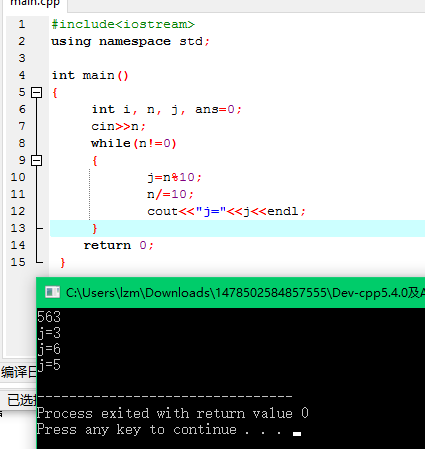# 蓝桥杯—平方怪圈 算法分析

1. #include<iostream>
2. using namespace std;
3. int main()
4. {
5.     int i, n, j, ans=0;
6.     cin>>n;
7.     for(i=0;i<50;i++)  //循环多少次 随便写
8.     {
9.
10.         while(n!=0)
11.         {
12.             j=n%10;
13.             ans+=j*j;
14.             n/=10;
15.         }
16.         printf(“ans=%d\n”,ans);
17.         n=ans;
18.         ans=0;
19.     }
20.
21.     return 0;
22.  }

1.         while(n!=0)
2.         {
3.             j=n%10;
4.             n/=10;
5.         }